### How to Add Two Images of Different Size in OpenCV Python?

In this post, we learn how to add two images of different sizes using OpenCV Python. We take two images of different sizes and perform pixel wise addition of these images. We use OpenCV Python method such as cv2.add(), cv2.addWeighted(), etc. to perform the operation.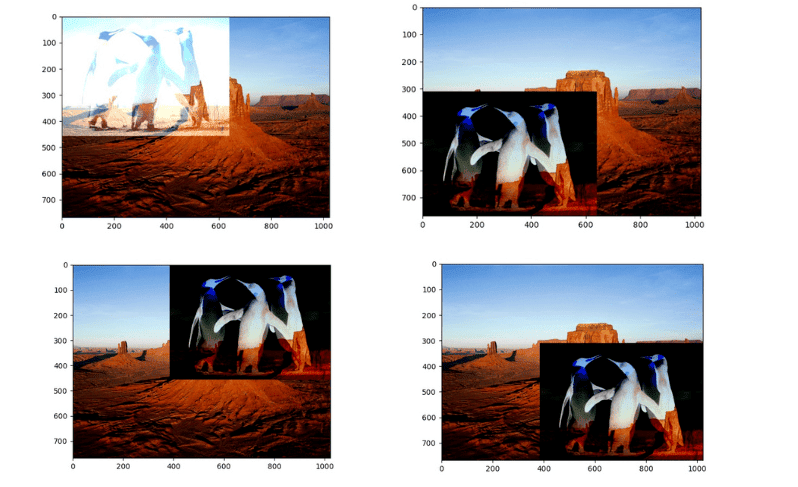Different Output Images after Adding Two Images

We will cover the contents listed in the following Table of Content.

Table of Content:

We will be using the following two images as the inputs to the programs discussed in this post.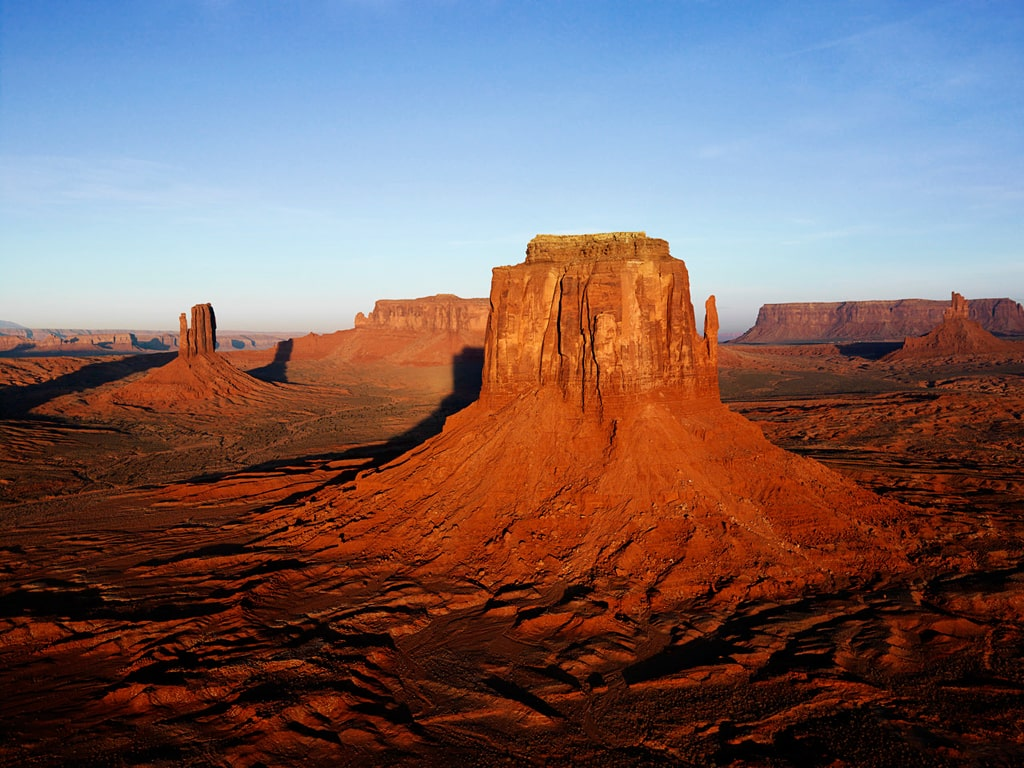Desert.jpgPenguins.jpg

Prerequisites
• Python 3
• OpenCV
• NumPy
• Matplotlib (Optional)

## Import Required Libraries/ Packages

The first step of any python programming is importing the required packages and libraries. We import the OpenCV (cv2) and Matplotlib. Using Matplotlib is optional. We used this to display the images with width and height.
`import cv2import matplotlib.pyplot as plt`

## Load and Visualize the Images

The next step is to load the images. We load to images "Desert.jpg" and "Penguins.jpg". You can download the images and follow the process with the same images

Here you should change the image path accordingly.
`# Load the input images img1 = cv2.imread('Desert.jpg')img2 = cv2.imread('Penguins.jpg')`
Now we should visualize the loaded image to make sure, we have loaded correct image. This part is optional.
`# Visualize the first imagecv2.imshow('added image',img1)cv2.waitKey(0)cv2.destroyAllWindows()`
Here we used OpenCV to display/ visualize the images.
To Visualize using Matplotlib follow the below two steps. Fist step is to convert images from BGR to RGB format and then visualize.
`# Convert them from BGR to RGBimg1 = cv2.cvtColor(img1, cv2.COLOR_BGR2RGB)img2 = cv2.cvtColor(img2, cv2.COLOR_BGR2RGB)`
Now visualize first image- Desert.jpg
`# Visualize First Imageplt.imshow(img1)plt.show()`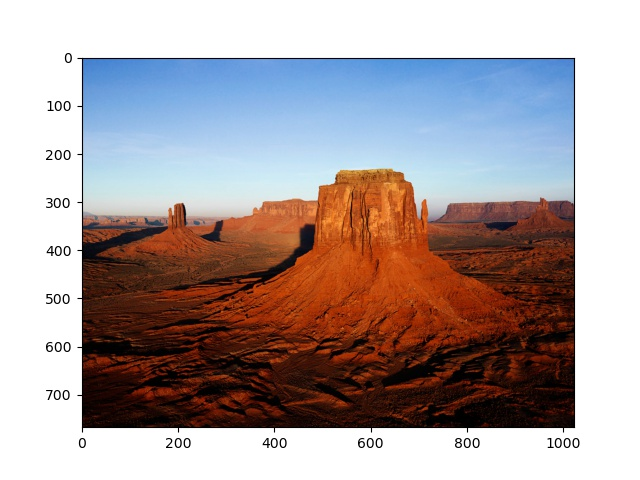Output- Desert.jpg

`# Visualize Second Imageplt.imshow(img2)plt.show()`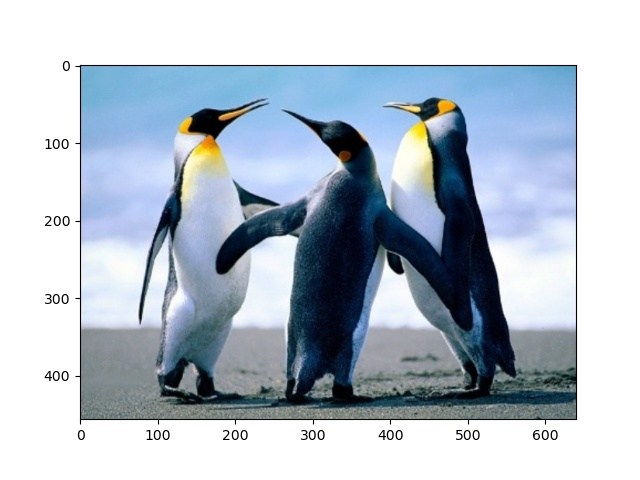Output -Penguins.jpg

We have discussed different ways to load image in Python in our previous post.

## Create ROI

The main task while adding or subtracting the images of different sizes is to find the ROI (Region Of Interest). Here our main aim is to find the minimum height and width from both the images. So that we can add the common area.

### Get height and width of images

First find the height and width of the images.
`# Get height and width of first imageheight1, width1 = img1.shape,img1.shapeprint(height1, width1)`
Output
768  1024

`# Get height and width of the second imageheight2, width2 = img2.shape,img2.shapeprint(height2, width2)`
Output
457  640

Notice the two images have different sizes (height and width).

### Find minimum height and width of images

Now find the minimum height and width of the images.
`# Find the minimum height and width of the two imagesmin_height = min(height1, height2)min_width = min(width1, width2)print(min_height, min_width)`
Output

457  640

### Crop images with minimum height and width

Crop both images with the above calculated minimum height and width.
`# Crop images with minimum height and widthimg11 = img1[0:min_height, 0:min_width]img22 = img2[0:min_height, 0:min_width]`
Now visualize the cropped images
`# Visualize first cropped imageplt.imshow(img11)plt.show()`
Output: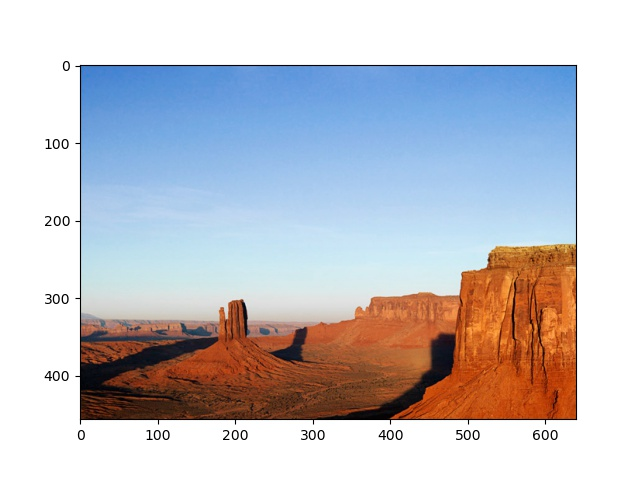Cropped image (Desert.jpg) with min height and width

Visualize second cropped image.
`plt.imshow(img22)plt.show()`
Output:Cropped image (Penguins.jpg) with min height and width

Now the both images have the same height and width. We could now add these images.

Note: You can OpenCV to visualize the output images. We are using the Matplotlib to show the scale for height and width.

### Create ROI by adding Cropped Images

Now add the cropped images with minimum height and width. This will be our ROI. We can use cv2.add() method to add the images of same size.
`# Creatte ROI by adding the cropped imagesimg_add = cv2.add(img11, img22)# Show the added miminum imagesplt.imshow(img_add)plt.show()`
Output: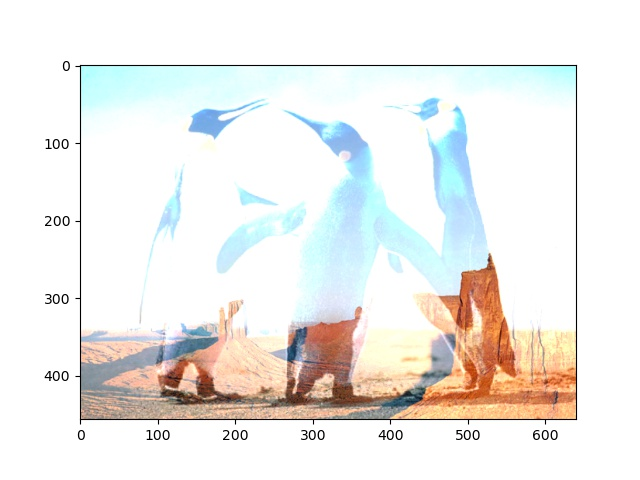ROI-image of min height and width

Alternatively you can use cv2.addWeighted() to add the images with different weights of images.
`# Add minimum images with different weightsimg_add = cv2.addWeighted(img11, 0.3, img22, 0.7, 0) plt.imshow(img_add)plt.show()`
We have added the common part of images. We can add this image part to the original images to find the final added images.

Let's now move to the final task to add the images. We add the cropped ROI to the original images.

### Finally add ROI to original Images

Using the indexing and slicing we add the ROI to the original images.
`img1[0:min_height,0:min_width] = img_addimg2[0:min_height,0:min_width] = img_add`

`plt.imshow(img1)plt.show()`
Output: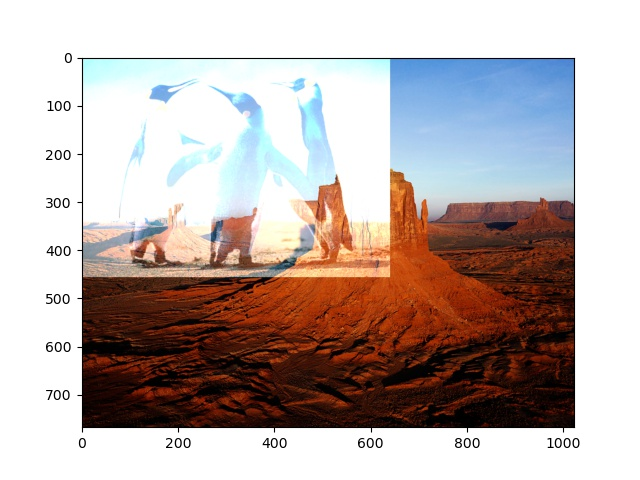Added Image (Image1+image2)

We have added the images. Notice the second image is added in the top-left part of the first image. Can you find the reason of this?
Can you add the second image image in bottom- right part of the first image or any other part?
For these questions look at FAQs at the end of the post.

See how the second image looks after adding.
`plt.imshow(img2)plt.show()`
Output:

## Complete Program 1 (add images of different sizes)

Now look at the complete program to add images of different size using OpenCV Python. The program describes the steps above discussed.
`# import required librariesimport cv2import matplotlib.pyplot as plt# Read two imagesimg1 = cv2.imread('Desert.jpg')img2 = cv2.imread('Penguins.jpg')def add_images(img1, img2):     height1, width1 = img1.shape,img1.shape    height2, width2 = img2.shape,img2.shape         min_height = min(height1, height2)    min_width = min(width1, width2)     img11 = img1[0:min_height, 0:min_width]    img22 = img2[0:min_height, 0:min_width]     img_add = cv2.add(img11, img22)      img1[0:min_height,0:min_width] = img_add    return img1img_add_result = add_images(img1, img2)# cv2.imshow('added image',img_add_result)# cv2.waitKey(0)# cv2.destroyAllWindows()# Convert the image from BGR to RGBimg_add_result = cv2.cvtColor(img_add_result, cv2.COLOR_BGR2RGB)plt.imshow(img_add_result)plt.show()`
Now look at some FAQS

## FAQs

### Q1. How to add smaller image at bottom-right part of bigger image.

Please make changes in the step- Finally add ROI to original Images. You can make indexing and slicing accordingly. Have a look at below code snippet.
`img1[height1-min_height: height1, width1-min_width:width1] = img_sub`
You can add this modified line in the complete program 1 and see how the output looks like.
`# import required librariesimport cv2import matplotlib.pyplot as plt# Read two imagesimg1 = cv2.imread('Desert.jpg')img2 = cv2.imread('Penguins.jpg')def subtract_images(img1, img2):        height1, width1 = img1.shape,img1.shape    height2, width2 = img2.shape,img2.shape        min_height = min(height1, height2)    min_width = min(width1, width2)        img11 = img1[0:min_height, 0:min_width]    img22 = img2[0:min_height, 0:min_width]        img_sub = cv2.subtract(img11, img22)            img1[height1-min_height: height1, width1-min_width:width1] = img_sub        return img1img_sub_result = subtract_images(img1, img2)cv2.imshow('Subtracted image',img_sub_result)cv2.waitKey(0)cv2.destroyAllWindows()# Convert the image from BGR to RGBimg_add_result = cv2.cvtColor(img_sub_result, cv2.COLOR_BGR2RGB)plt.imshow(img_add_result)plt.show()`
Output:

### Q2. How to add smaller image at bottom-left part of bigger image.

As in the first Question, please make changes in the step- Finally add ROI to original Images. You can make indexing and slicing accordingly. Have a look at below code snippet.
`img1[height1-min_height: height1, 0:min_width] = img_sub`
You can add this modified line in the complete program 1 and see how the output looks like.

### Q3. How to add smaller image at left-bottom part of bigger image.

As in the first Question, please make changes in the step- Finally add ROI to original Images. You can make indexing and slicing accordingly. Have a look at below code snippet.
`img1[0:min_height, width1-min_width:width1] = img_sub`
You can add this modified line in the complete program 1 and see how the output looks like.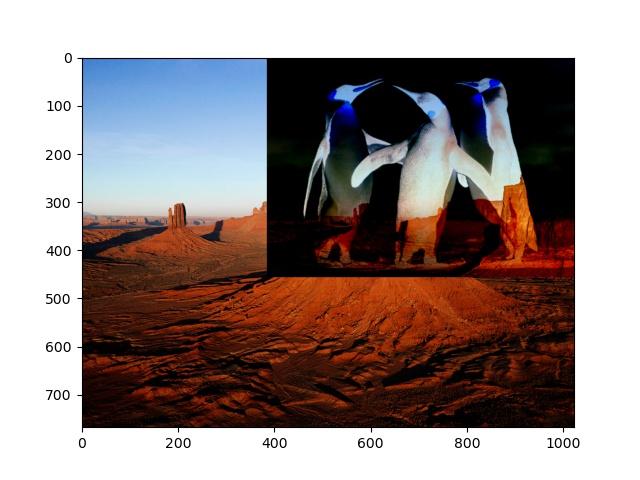Added image at top-right

We have covered all the content related to adding and subtracting images of different sizes ( different height and width). If you have any doubts or want more related to this topic please comment.